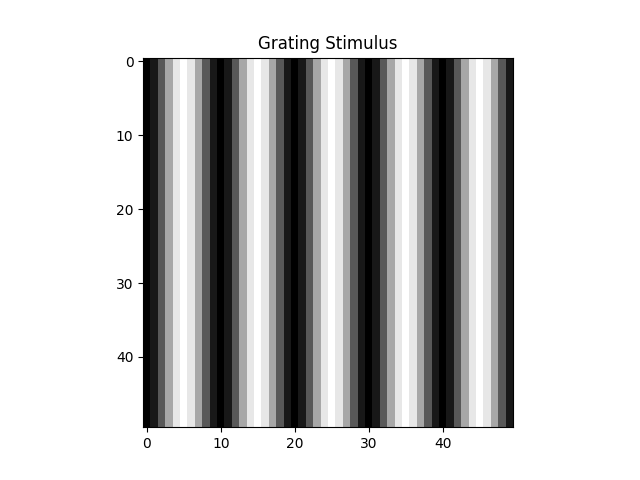# Generating a drifting sinusoidal grating or drifting bar stimulus¶

This example shows how to use drifting psychophysics-based stimuli for a retinal implant.

Along with images, videos, and oter built-in stimuli, pulse2percept supports generating GratingStimulus and BarStimulus as stimuli that can be passed as percepts to implants.

## Creating a Stimulus¶

First, create the stimuli:

Shape ((height, width) in pixels) is the only required parameter for creating these stimuli.

A drifting sinusoidal grating is represented by GratingStimulus. The following illustrates one frame of a grating stimulus.

import matplotlib.pyplot as plt
from pulse2percept.stimuli import GratingStimulus
stim = GratingStimulus((50, 50), spatial_freq=0.1, temporal_freq=0.1)
plt.imshow(stim.data[:, 0].reshape(50, 50), cmap='gray')
plt.title("Grating Stimulus")
plt.show()You can view the entire stimulus over time using stim.play()

stim.play()


Here, the spatial frequency of the grating (i.e., the inverse of how many pixels it takes to represent one cycle of the sinusoid) is given as 0.1 cycles/pixel, whereas the temporal frequency (i.e., the inverse of how many frames it takes to represent one cycle of the sinusoid) is given as 0.1 cycles/frame. By default, the drift direction of the grating will be to the right (0 degrees).

A drifting bar is represented by BarStimulus. A visual example of a basic sinusoidal grating can be generated as such:

from pulse2percept.stimuli.psychophysics import BarStimulus
stim = BarStimulus((50, 50), speed=1)
stim.play()